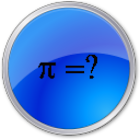Math Quiz High School

All Android applications categories

All Android games categories# Math Quiz High School

by: 1 4

4 Users
rating

## Screenshots

Description

This math app aimed at senior high school (can also be used at first year in college) is a fun way to practice your math skills and check how much you know. It's made as quiz with 7 different categories where you can try your skills.

There is also a high score list so you can keep track of which categories where you might need to work on the material and which you are doing great! Many of the questions also have small explanations to help you out if you answer wrongly.

The questions are chosen randomly each time so you can try out the same category a few times and still get new questions in mathematics.

There is also a search function so you can use it as a math reference as well as a quiz.

The categories included are:
Differential calculus
Integrals
Vectors in 2D and 3D
Geometry in 2D and 3D
Functions of 1 variable (f(x) = ..)
Vector funtions
Differential equations.

Have Fun and learn!
(note: this app is only in English)

Tags: mathquiz la pantalla , first year high school quiz sheet , quiz math , math quizzes for high school , u torrent math quiz , sample math quiz for high school , quiz for math torrent , pondar apk las aberturas del ajedrez torrent , mathematics college quiz apk

from 1 reviews

"OK"

4